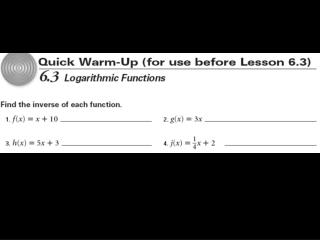DownloadDownload PresentationLogarithms are used to find unknown exponents in exponential models.

# Logarithms are used to find unknown exponents in exponential models.

Télécharger la présentation## Logarithms are used to find unknown exponents in exponential models.

- - - - - - - - - - - - - - - - - - - - - - - - - - - E N D - - - - - - - - - - - - - - - - - - - - - - - - - - -
##### Presentation Transcript

1. 6. 3 Logarithmic FunctionsObjectives: Write equivalent forms for exponential and logarithmic equations.Use the definitions of exponential and logarithmic functions to solve equations.Standard: 2.8.11.S. Analyze properties and relationships of functions.

2. Logarithms are used to find unknown exponents in exponential models. Logarithmicfunctions define many measurement scales in the sciences, including the pH, decibel, and Richter scales.

3. With logarithms, you can write an exponential equation in an equivalent logarithmic form.

4. Ex 1. a. Write in logarithmic form. ________________________ b. Write in exponential form. _______________________ c. Write 112 = 121 in logarithmic form. _________________________ d. Write log 6 36 = 2 in exponential form. _______________________ e. Write 7-2=1/49 in logarithmic form. __________________________ f. Write log 3 1/81= -4 in exponential form. _______________________ 2 = log11 121 62 = 36 Log7 (1/49) = -2 3-4 = 1/81

5. You can evaluate logarithms with a base of 10 by using the log key on a calculator. Ex 2. Solve each equation for x. Round your answer to the nearest thousandth. a). 10x= 1/109 b). x = log101/109 x = -2.037 c). 10x= 1.498 d). 10x= 7210 x = log10 1.498 x = log107210 x = .176 x = 3.858

6. The inverse of the exponential function y = 10x is x = 10y. To rewrite x = 10y in terms of y, use the equivalent logarithmic form, y = log 10 x.

7. Examine the tables & graphs below to see the inverse relationship between y=10x and y = log10x. y= 10x y=x y = log10x

8. BeBelow summarizes the relationship between the domain and range of y = 10x and of y = log10 X. • y = 10x Domain: all Real #s Range: all positive Real #s • y = log10 X Domain: all positive real #s Range: all Real #s

9. The logarithmic function y = log b x with base b, or x = by, is the inverse of the exponential function y = bx, where b ≠ 1 and b > 0. One-to-one Property of Exponents If bx = by, then x = y.

10. Ex. 3 Find the value of v in each equation. A. B. 5 = logv 32 v = log125 5 v5 = 32 125v = 5 (53)v = 5 v5 = 25 53v = 51 v = 2 3v = 1 v = 1/3

11. d. v = log464 4v = 64 4v = 43 (same base) v = 3 c. 4 = log3 v 34 = v 81 = v

12. g. v = log10 1000 10v = 1000 10v = 103 v = 3 e. 2 = logv25 v2 = 25 v2=52 v = 5 h. 2 = log7V V = 72 V = 49 f. 6 = log3v v = 36 v = 729 I.1 = log3v 31 = v 3 = v

13. Homework Integrated Algebra II- Section 6.3 Level A Honors Algebra II- Section 6.3 Level B Read article and write one paragraph on your thoughts as they relate to the exponential growth of money